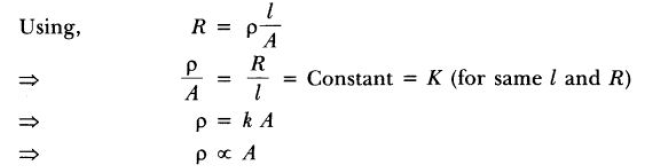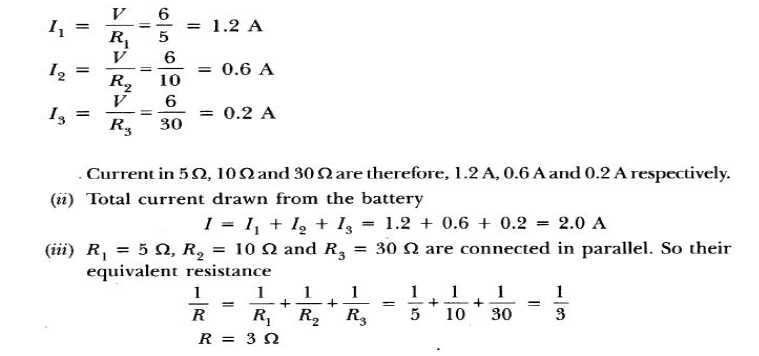# CBSE Class 10 Science Electricity Assignment Set C

Read and download free pdf of CBSE Class 10 Science Electricity Assignment Set C. Get printable school Assignments for Class 10 Science. Standard 10 students should practise questions and answers given here for Chapter 12 Electricity Science in Grade 10 which will help them to strengthen their understanding of all important topics. Students should also download free pdf of Printable Worksheets for Class 10 Science prepared as per the latest books and syllabus issued by NCERT, CBSE, KVS and do problems daily to score better marks in tests and examinations

## Assignment for Class 10 Science Chapter 12 Electricity

Class 10 Science students should refer to the following printable assignment in Pdf for Chapter 12 Electricity in standard 10. This test paper with questions and answers for Grade 10 Science will be very useful for exams and help you to score good marks

### Chapter 12 Electricity Class 10 Science Assignment

Question : Which of the following terms does not represent electrical power in a circuit?
a. I2R
b. IR2
c. VI
d. V2/ R

Question :  The following instruments are available in a laboratory :Milliammeter A1 of range 0- 300 mA and least count 10 mAMilliammeter A2 of range 0-200 mA and least count 20 mAVoltmeter V1 of range 0-5 V and least count 0.2 VVoltmeter V2 of range 0-3 V nd least count 0.3 V. Out of the following pairs of instruments, which pair would be the best choice for carrying out the experiment to determine the equivalent resistance of two resistors connected in series ? (1)
a. Milliammeter A2 and voltmeter V2
b. Milliammeter A1 and voltmeter V1
c. Milliammeter A2 and voltmeter V1
d. Milliammeter A1 and voltmeter V2

Explanation: Milliammeter A1 and voltmeter V1 gives maximum measuring range with lowest least count. So, the combination of these two is best for two resistances connected in series.

Question : What is the resistivity of Nichrome?
a. 110 x 10-8Ω m
b. 12.9 x 10-8 Ω m
c. 1010 - 1014 Ω m
d. 1.60 x 10-8 Ω m

Question : Using the adjoining circuit, current and potential difference are measured and plotted in a graph. The best suited graph isQuestion : Name a device that helps to maintain a potential difference across a conductor.

Question : Two resistors of 10 Ω and 15 Ω are connected in series to a battery of 6 V. How can the values of current passing through them be compared?
Answer :  In series, same current flows through each resistor. So, ratio of current is 1: 1.

Question. What is meant by saying that the potential difference between two points is 1 V?
Answer: The potential difference between two points is said to be 1 volt if 1 joule of work is done in moving 1 coulomb of electric charge from one point to the other.

Question. Calculate the least count of voltmeter in which there are 10 divisions between 1.0 V and 1.5 V marks.
Answer: Least count = 0.5/10 = 0.05.

Question. Would you connect a fuse in series or in parallel to an electric circuit ?
Answer: In series, of the electric circuit before appliances are present in the circuit.

Question. Why do electricians wear rubber hand gloves while working ?
Answer: Rubber is an electrical insulator. Hence, electrician can work safely while working on an electric circuit without a risk of getting any electric shock.

Question. An electric heater is rated ‘1500 W, 250 V’. The heater is connected to 250 V mains. Calculate :
(a) The current drawn.
(b) The energy consumed in 50 hours.
(c) The cost of energy consumed at ₹6 per kWh.
Answer: (a) 6A, (b) 75 kWh, (c) Rs. 450.

Question. Which substance is used for making resistance coil of electric heater and why ?
Answer: Nichrome, due to its high resistivity.

Question. What is commercial units of electrical energy ? Convert it into joules.
Answer: The commercial unit of electrical energy is kWh.
1 kWh = 1000 W × 1 hour
= 1000 J/s × 60 × 60 s
= 3.6 × 106 J

Question. Name and define the S.I. unit of electric power.
Answer: The S.I. unit of electric power is watt (W). One watt is the power consumed by an electrical device when it is operated at a potential difference of one volt and carries a current of one ampere.
1 watt = 1 volt × 1 ampere.

Question. Why is an ammeter connected in series in an electric circuit ?
Answer: It is connected in series so that whole of electric current, which it has to measure, passes through it.

Question. A boy noted the readings of his home’s electricity meter on 1st June and again on 1st July (as shown in figure given below) :
(a) What was the meter reading on 1st June ?
(b) What was the meter reading on 1st July ?
(c) How many units of electrical energy have been used ?
(d) Calculate the cost of electrical energy used, if the rate is Rs.
5 per unit.
(b) 67912
(c) 310
(d) Rs. 1550.

Question. In an activity performed by a girl to estimate the monthly bill of her house, she reported that 3 bulbs of 100 W each, 2 fans of 50 W each and 1 T.V. set of 60 W are used daily for an average of 8 hours, 10 hours and 5 hours respectively.
(a) Calculate the electrical energy (in kWh) consumed in 1 month.
(b) If the cost of electrical energy is R 6 per unit, what is the monthly bill ?
(b) ₹ 666.

Question. Is Joule heating always desirable ?

Question. Write the function of voltmeter in an electric circuit.
Answer: Voltmeter measures the potential difference across in a circuit. It is always connected in parallel in the circuit.

Question. Write two points of difference between electrical energy and electric power.Question :  Define the term "volt".
Answer :  The potential difference between two points A and B is said to be one volt if 1 joule of work is done to move 1 coulomb of charge from one point to another point in an electric field.

Question : What constitutes the current ?
Answer :  The flow of electric charges across a cross-section of a conductor constitutes an electric current. For example, a stream of electrons moving through a conducting wire constitutes an electric current.

Question. Name the practical unit of power and state its relation with the S.I. unit.
Answer: The practical unit of power is Horse Power (H.P.)
1 H.P. = 746 watt.

Question. Write the formula of electric power (P) in terms of :
(a) Potential difference (V) and current (I).
(b) Current (I) and resistance (R).
(c) Potential difference (V) and resistance (R).
(b) P = I2R
(c) P = V2/R

Question. What will happen when :
(a) Voltmeter is connected in series?
(b) Ammeter is connected in parallel?
Answer: (a) Negligible current will pass through the circuit because the voltmeter has a very high resistance.
(b) Ammeter will get damaged due to flow of large amount of current through it, because it has low resistance.

(a) What is an ammeter?
(b) What is a voltmeter?
(c) Define resistivity.
Answer: (a) Ammeter is an instrument which is used to measure electric current in a circuit. It is always connected in series with the circuit.
(b) A voltmeter is an instrument which is used to measure electric potential in the circuit between two points. It is always connected in parallel with the circuit.
(b) The resistivity of a substance is numerically equal to the resistance of a rod of that substance which is one metre long and one square metre in cross-section.

Question. Why is the tungsten metal more coiled in the bulb and not installed in straight p arallel wire form?
Answer: The coiled wire of tungsten increases the surface area of the wire in very less space so as to emit more light and helps in glowing with more intensity.

Question. What would you suggest to a student if while performing an experiment he finds that the pointer/needle of the ammeter and voltmeter do not coincide with the zero marks on the scales when circuit is open? No extra ammeter/voltmeter is available in the laboratory.
Answer: This is called the zero error of the scale of ammeter or voltmeter. If there is a zero error then this error is subtracted from the value that depicts when the circuit is closed otherwise accurate reading will not be recorded.

Question. Why we must keep the circuit closed for a relatively shorter time and open for a relatively longer time to ensure minimal changes in the values of resistance?
Answer: Due to heating effect of current, we must keep the circuit closed for a relatively shorter time and open for a relatively longer time to ensure minimal changes in the values of resistance.

Question. A torch bulb when cold has a resistance of 2·5 W. It draws a current 450 mA, when connected to a 6 V battery and glows brightly. Calculate the resistance of the bulb when glowing and explain the reason for the difference in resistance.
Answer: While glowing, I = 450 mA = 0.45 A, V = 6 volt
Using Ohm’s law, Resistance of bulb, R = V/1 = 6/0.45 = 13.33 W
The reason for the difference in resistance of bulb when cold (R = 2·5 W) and while glowing (R = 13·33 W), is that the resistance of filament of bulb increases with the increase in temperature.

Question. A uniform wire with a resistance of 32 W is divided into four equal parts and they are joined in parallel. Calculate the equivalent resistance of the parallel combination.
R1 = 32/4 W = 8 W
When connected in parallel, the equivalent resistance is
1/R = 1/8 + 1/8 + 1/8 +1/8 = 4/8 = 1/2
or R = 2 W.

Question. What do you mean by an electric circuit ? Carefully study the circuit diagram given below and make the necessary corrections.Answer: An electrical circuit is a continuous path comprising conducting wires and other electrical components between the terminals of a battery along which an electric current is set up. It is represented by drawing a circuit diagram. The corrected circuit diagram given in the question is :Question.The given figure shows the V-I graphs for two resistors. Identify the resistor that obeys Ohm’s law. Give a reason for your answer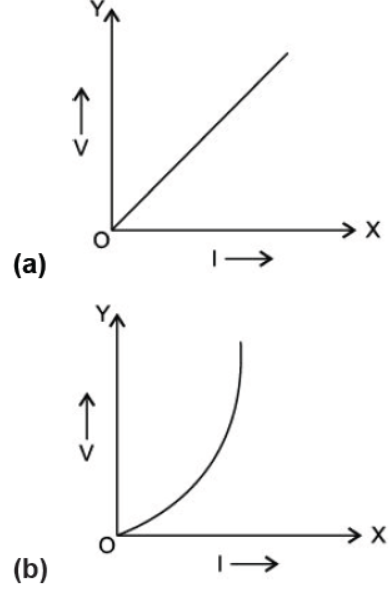Answer: The figure (a) obeys Ohm’s law because the V-I graph is a straight line and its slope (or resistance) is constant.

Question :  How many bulbs of 8 should be joined in parallel to draw a current of 2A from a battery of 4 V?
Given,

current ( i ) = 2 A

voltage ( v ) = 4 V

Resistance of bulb is R1 = 8Ω

resistance ( R ) = ?Question :  Radhika is a student of class X. Her mother was making tea in an old electric kettle having metal case. When she switched on the power supply to the electric kettle. She got a severe electric shock. Radhika put off the main switch quickly and found that the connecting cord was torn, where her mother touched the metal case of the kettle.
She also found that the red and black wires of connecting cord were firmly connected to the two lower terminals of the power plug but the green wire of cord was not connected to the upper terminal of the plug. Radhika replaced the torn connecting cord and also connected to the three wires of cord firmly to the power plug terminals.
On the basis of the above passage, answer the following questions:
i. Why did Radhika put off the main switch quickly?
ii. Which wire red, black or green, touched the metal case of electric kettle when Radhika's mother got electric shock?
iii. What values are displayed by Radhika in this incident?
i. Radhika put off the main switch quickly to save her mother.

ii. Red wire which is at a high potential of  220V was touching the metal case of electric kettle.

iii. The values displayed by Radhika are:

a. Concern for her mother.

b. Presence of mind.

c. Knowledge of household wiring and daily life activities.

Question : What would you suggest to a student if  while performing an experiment he finds that the pointer hneedle of the ammeter and voltmeter do not coincide with the zero marks on the scales when circuit is open? No extra ammeter  voltmeter is available in the laboratory.
Answer :  As pointer of both the ammeter and voltmeter do not coincide with the zero marks on the scales when circuit is open, it indicates zero error in both the instruments. This zero error should be subtracted from the readings taken when circuit is closed. o get correct readings using these instruments, first we should find out the Least counts of both the nstruments, i.e., the minimum value which can be accurately measured.
Zero error = Initial reading (in open circuit) = Pointer reading (in open circuit) X Least count To get the actual reading, subtract zero error or the initial reading of the open circuit to the reading when you perform the experiment. Actual reading = Final reading - Initial reading

Question :  What is meant by resistance of a conductor? Name and define its SI unit. List the factors on Which the resistance of a wire affected, if (i) its length is doubled, (ii) its radius is doubled?
Answer : Property to oppose the flow of electric current is called resistance. Its SI unit is ohms.
If 1V potential difference is there and 1A current is flowing then it is said that there is 1 ohm resistance.Thus, resistance will decrease by four times, if radius of wire is doubled.

Question : (A) Define electrical energy with S.I. unit?
(B) A household uses the following electric appliance;
(i) Refrigerator of rating 400w for ten hour each day.
(ii) Two electric fans of rating 80w each for twelve hours each day.
(iii) Six electric tubes of rating 15w each for six hours each day.
Calculate the electricity bill of the household for the month of june if the cost per unit of electric energy is ` 3.00.
Answer :  (A) The work done by a source of electricity to maintain current in a circuit is known as electrical energy. Its S.I. unit is Joule.
(B) (i) Electricity consumed by refrigerator = Power × Time
= 400 × 10
= 4000 Wh
= 4 kWh
(ii) Electricity consumed by two fans
= Power × Time
= 80 × 2 × 12
= 1920 Wh
= 1.92 kWh
(iii) Electricity consumed by six electric tubes
= 6 × 18 × 6
= 648 Wh
= 0.648 kWh
Total energy consumed in one day
= 4 + 1.92 + 0.648
= 6.548 kWh
Total energy consumed in one month
= 6.548 × 30
= 197.04 kWh
Cost of 1 unit (kWh)                                 = R 3.00
Cost of 197.04 kWh                                  = 197.04 × 3.00
Total electricity bill                                    = R 591.12

Question : How much current will an electric bulb draw from 220 V source if the resistance of the bulb is 1200Ω? If in place of bulb, a heater of resistance 100 Ω is connected to the sources, calculate the current drawn by it.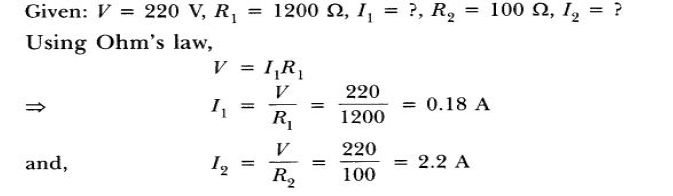Question : Out of the two wires X and Y shown below, which one has greater resistance? Justify your answer.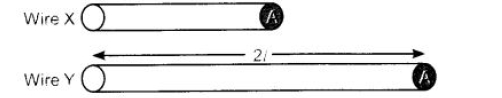Answer :  Wire ‘Y’ has greater resistance as it has more length than wire ‘X’. It is because resistance of wire is directly proportional to the length of wire.

Question : The figure below shows three cylindrical copper conductors along with their face areas and lengths. Discuss in which geometrical shape the resistance will be highest.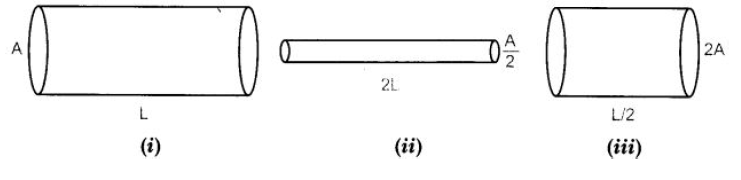Question : Two wires A and B are of equal length and have equal resistance. If the resistivity of A is more than that of B, which wire is thicker and why? For the electric circuit given below calculate: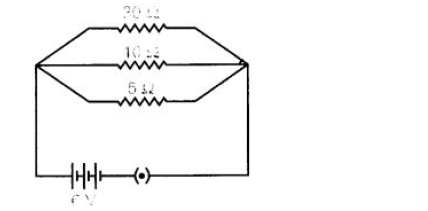(i) Current in each resistor,
(ii) Total current drawn from the battery, and
(iii) Equivalent resistance of the Circuit